# Hidden constant factor

(Redirected from Invisible constant factor)

When the time or space required for an algorithm is expressed in terms of the input size using big O notation, constant factors are destroyed. For example, if one algorithm requires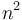$n^2$ nanoseconds on a given machine, and another requires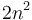$2n^2$ nanoseconds on that machine, then both algorithms are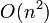$O(n^2)$, and from that information alone we cannot determine that the latter is faster than the former. That is, in big O notation, the constant factor is hidden.

From a theoretical point of view, this is advantageous, since we could always design a faster machine, which would make our algorithms take less time to run, but that wouldn't reflect the efficiency of the algorithm itself; and so we always want to discard the constant factor. In practice, however, the hidden constant factor is very important. If one algorithm requires$n^2$ nanoseconds and another requires$n$ milliseconds, then the latter appears to be more efficient as it is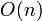$O(n)$ rather than$O(n^2)$, but in practice is only faster when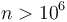$n > 10^6$.

The discrepancy in hidden constant factor between two algorithms with the same asymptotic running time (big O) is a consequence of three main factors:

• Some algorithms, by nature, simply require more operations than others. Bubble sort, for example, tends to use more operations than insertion sort. Bubble sort can only reorder elements by swapping two adjacent elements at a time, and swapping two elements requires three copy operations (as an intermediate variable has to be used), and swapping two elements eliminates an inversion from the sequence. On the other hand, insertion sort moves elements longer distances at once. When an element is moved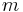$m$ positions, it eliminates$m$ inversions, and requires$m+2$ copy operations; and thus will insertion sort will generally average a bit more than one copy operation per inversion.
• Some operations are slower than others. For example, multiplication and division of floating point numbers tends to be slower than addition and subtraction. Thus, for example, if a primitive in computational geometry can be implemented using either six additions and two multiplications or four additions and three multiplications, both implementations take constant time, but the former is probably faster.
• Some algorithms exhibit better locality of reference than others. For example, two nested for loops that iterate over a two-dimensional array should always be written so that they access the elements of the array in sequence in RAM, rather than in the other order. For example, in C this means that they should access elements in the other `A, A, A, ..., A, ...` rather than in the order `A, A, A, ..., A, ...`. The former hits the cache on almost every access; the latter always misses it.

Here are some general useful conclusions that can be drawn about the hidden constant factor:

• Quicksort is generally faster than heapsort and mergesort, though each has average-case performance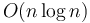$O(n \log n)$. Furthermore, most programming language standard libraries include highly optimized sorting routines. The$O(n \log n)$ time required to sort generally has a lower constant factor than almost any other$O(n \log n)$ algorithm you might want to implement for the same input.
• The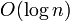$O(\log n)$ time associated with a binary heap operation generally has lower constant factor (is faster) than the$O(\log n)$ time associated with a balanced binary search tree operation. Thus, BBSTs implement a superset of the functionality of heaps, but at the cost of slower running time.
• Segment trees require about twice as much memory as binary indexed trees (and incur an additional factor of 2 for each additional dimension), and an$O(\log n)$ segment tree operation is generally slower than an$O(\log n)$ BIT operation, too.
• Suffix trees use more memory and take more time to construct than suffix arrays, though both are linear.